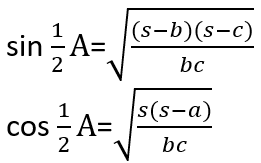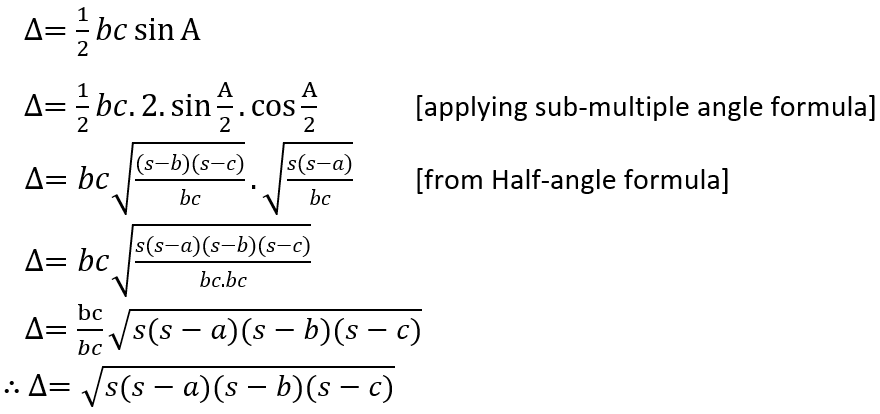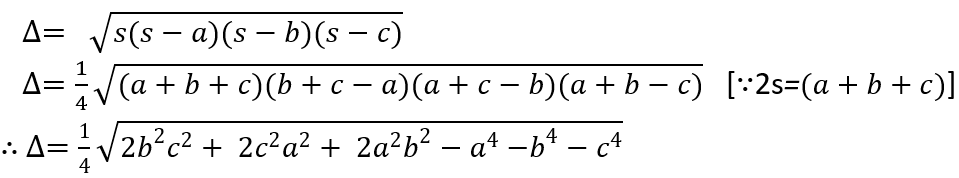The Area of TriangleThe area of triangle ABC with the given measurement as shown in fig is ''. 's' is the semi-perimeter of the triangle such that 2s=().

We know, area of triangle is given by;From Half-angle formula, we have,1st Proof:2nd Proof:
We have,3rd Proof: